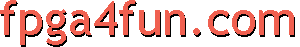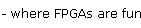## VHDL tips and tricks

This page is not really a tutorial, but a list of sample code that you can study and refer to when you start writing VHDL.

Thanks for Timothy Dahlin for his contribution.

##### Bit operations
```library IEEE;
use IEEE.STD_LOGIC_1164.ALL;
use IEEE.STD_LOGIC_ARITH.ALL;
use IEEE.STD_LOGIC_UNSIGNED.ALL;

entity bitops is
Port( A :  in std_logic_vector(3 downto 0);
B :  in std_logic_vector(3 downto 0);
C : out std_logic_vector(3 downto 0);
D : out std_logic_vector(3 downto 0);
E : out std_logic_vector(3 downto 0);
F : out std_logic_vector(3 downto 0);
G : out std_logic_vector(3 downto 0);
H : out std_logic_vector(3 downto 0);
I : out std_logic_vector(3 downto 0);
J : out std_logic_vector(3 downto 0);
K : out std_logic_vector(3 downto 0)
);
end bitops;

architecture Behavioral of bitops is
begin
C <= A and B;
D <= A xor B;
E <= not A;
F <= A or B;
G <= A nor B;
H <= A nand B;
I <= A xnor B;
J <= B(3) & A(1 downto 0) & '1';    -- concatentation
K <= B(2 downto 1) & B(2 downto 1);    -- replication
end Behavioral;
```
##### 2-to-1 mux
```library IEEE;
use IEEE.STD_LOGIC_1164.ALL;
use IEEE.STD_LOGIC_ARITH.ALL;
use IEEE.STD_LOGIC_UNSIGNED.ALL;

entity mux2t1 is
Port ( A : in std_logic;
B : in std_logic;
S : in std_logic;
Y : out std_logic);
end mux2t1;

architecture Behavioral1 of mux2t1 is
begin
process(A, B, S)
begin
if(S = '1') then
Y <= A;
else
Y <= B;
end if;
end process;
end Behavioral1;

architecture Behavioral2 of mux2t1 is
begin
process(A, B, S)
begin
case S is
when '1' =>
Y <= A;
when others=>
Y <= B;
end case;
end process;
end Behavioral2;

architecture Behavioral3 of mux2t1 is
begin
Y <= A when (S = '1') else B;
end Behavioral3;

architecture Behavioral4 of mux2t1 is
begin
with S select
Y <= A when '1',
B when others;
end Behavioral4;
```